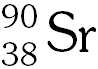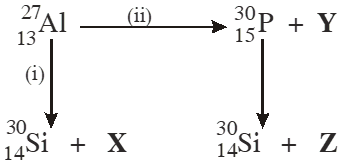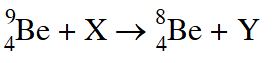Download the eSaral app and start learning from Kota's top IITians and doctors.

JEE Advanced Previous Year Questions of Chemistry with Solutions are available at eSaral. Practicing JEE Advanced Previous Year Papers Questions of Chemistry will help the JEE aspirants in realizing the question pattern as well as help in analyzing weak & strong areas. Simulator Previous Years JEE Advance Questions
Q. The total number of $\alpha$ and $\beta$particles emitted in the nuclear reaction $_{92}^{238} \mathrm{U} \rightarrow_{82}^{214} \mathrm{Pb}$ is [JEE 2009]
Ans. 8
Q. The number of neutrons emitted when $_{92}^{235} \mathrm{U}$ undergoes controlled nuclear fission to $_{54}^{142} \mathrm{Xe}$ andis - [JEE 2010]
Ans. 4
Q. Bombardment of aluminium by $\alpha$ -particle leads to its artificial disintegration in two ways, (i) and (ii) as shown. Products X, Y and Z respectively are :(A) proton, neutron, positron (B) neutron, positron, proton (C) proton, positron, neutron (D) positron, proton, neutron [JEE 2011]
Ans. (A)
Q. The periodic table consists of 18 groups. An isotope of copper, on bombardment with protons, undergoes a nuclear reaction yielding element X as shown below. To which group , element X belongs in the periodic table ? [JEE 2012]
Ans. 8
Q. In the nuclear transmutation(X, Y) is(are) $(\mathrm{A})(\gamma, \mathrm{n})$ (B) (p, D) (C) (n, D) $(\mathrm{D})(\gamma, \mathrm{D})$ [JEE 2013]
Ans. (AB)
Q. A closed vessel with rigid walls contains 1 mol of 238 92 $\mathrm{U}$ U and 1 mol of air at 298 K. Considering complete decay of $^{238}_{92} \mathrm{U}$ the ratio of the final pressure to the initial pressure of the system at 298 K is - [JEE 2015]
Ans. 9